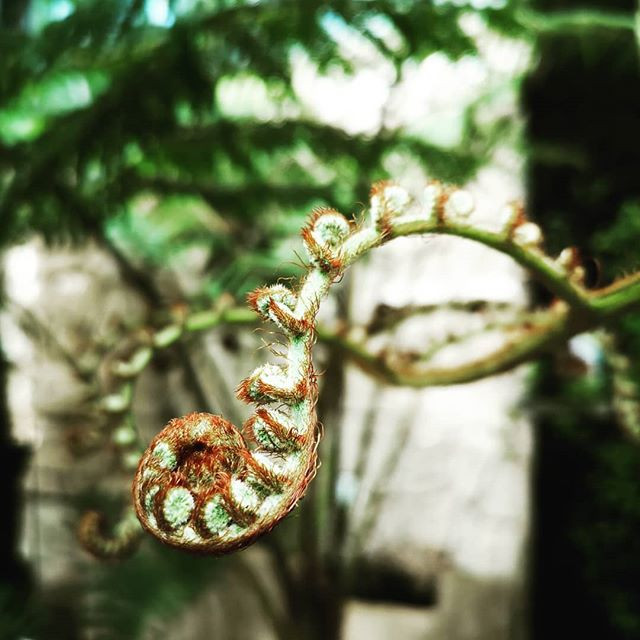Search
• Prisca

# What does a number sequence and a daisy have in common?

Updated: Mar 3, 2019

The Fibonacci sequence is a sequence of numbers created by adding up the two preceding ones: 0, 1, 1, 2, 3, 5, 8, 13, 21, 34 and so on (which means forever). The famous known Fibonacci Spiral is the graphical depiction of this sequence:But what does this mathematical number sequence have to do with plants or nature in general? In fact, this simple pattern is appearing to you on a daily basis. You probably just didn't notice yet. All the chaos, the colours and the beauty of nature can be captured by the Fibonacci Sequence.

The most famous example is the number of petals of flowers. They almost always follow the Fibonacci Sequence. Strawberries (the plants I am working with) have five petals. Lily’s show 3 and some daisies have 34 petals. Bu there is even more: Spiraling patterns appear in seed arrangement, pine cone formation, in the way how ferns curl or leaf arrangements around a stem.Can you see the spirals?

Have you ever heard of the Golden Ratio? The Golden Ratio is connected to the Fibonacci Sequence. If numbers of the Fibonacci Sequence are divided by their previous one, you are getting the following result:

1:1 = 1

2:1 = 2

3:2 = 1,5

5:3 = 1,666

8:5 = 1,6

13:8 = 1,625

21:13 = 1,615

As you might have noticed: the results are staying around the number 1,6. The golden Ratio or Golden Number (also called phi) is: 1,618. Interestingly, we define beauty by the Golden Ratio (1:1,618). The closer proportions in a human face come to this ratio, the more beautiful it appears to us. Examples are: the ratio of the width of the eye to the width of the iris or the outer and inner edge of the eye to the center of the nose.

I am simply fascinated by the fact, that you can squeeze something so vast and chaotic in a strict mathematical formula. That even beauty and attractiveness falls under this rule and that is seems like as if you can cage nature itself with a simple sequence of numbers. I thought this short excursion into math was worth sharing even as a plant science related blog.See All